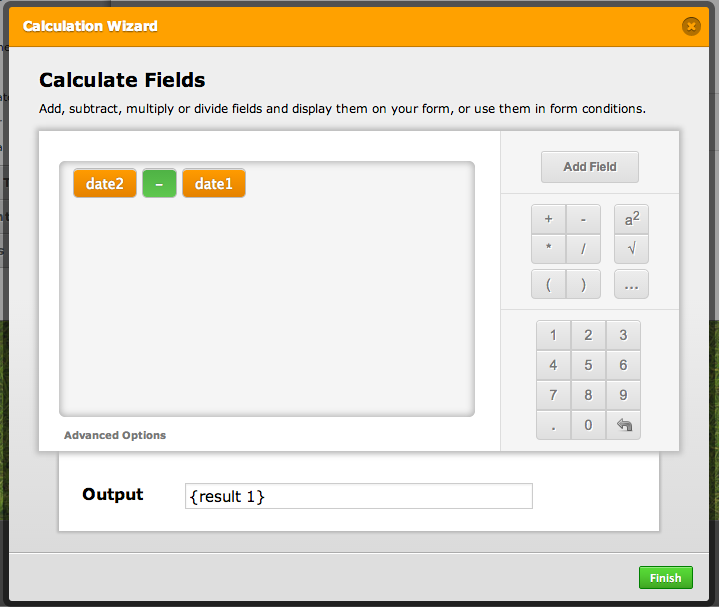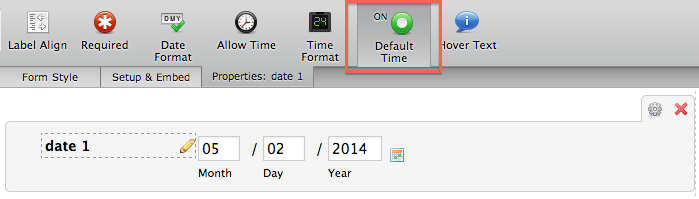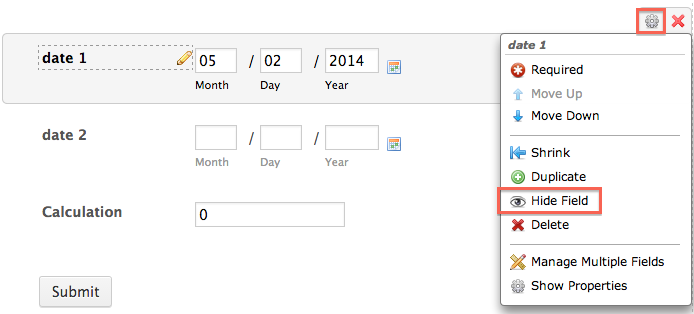# Can I calculate the number of days between two dates (example: Today mi

•demnos
Asked on May 02, 2014 at 03:35 AM

Can I calculate the number of days between two dates (example: Today minus a date from my form)?

This is a re-post of a comment on How to Perform Form Calculation Using a Widget

•ceren
Answered on May 02, 2014 at 04:14 AM

Hi demnos,

You can achieve your requirement with using our Form Calculation widget.

First you need to add 2 date fields to your form. Then add Form Calculation widget and configure it to calculate the number between two dates.If you want to calculate the difference between today and the other date field you can set first date field’s value to default and hide it. This will set date field value to today and it will never be seen on your form.If you have any other questions, please let us know.

•demnos
Answered on May 03, 2014 at 05:29 PM

I'll give this a try.  Thanks!

•demnos
Answered on May 03, 2014 at 05:47 PM

Ok, so this calculation isn't working.

I tried to calculate May 3, 2014 (today) - May 1, 1981 I get an answer of 4212.7.  What does this number represent?  It isn't days because if you divide it by 365 (days in a year) you get 11.54 when I would expect something around 33 years.  Do you know how this application handles dates?

•Welvin
Answered on May 03, 2014 at 09:51 PM

You have to disable the TIME in the DateTime field so to have the correct days. To disable, simply click on the "Allow Time" button:Example Form: http://www.jotformpro.com/form/41228066850958

Thanks

•demnos
Answered on May 03, 2014 at 10:14 PM

Got it working then.  Thanks.

I was using the Birthday Picker instead of the plain DateTime picker.  :-(

•himanshugogia
Answered on May 10, 2016 at 08:50 AM

Hey,

I need to calculate number of days between two dates and the result shown should exclude Saturday and Sunday.

•